## Theory of Combinatorial Algorithms

Prof. Emo Welzl and Prof. Bernd Gärtner

Satisfiablity (SAT) Course, 2005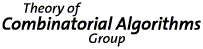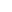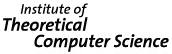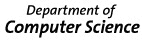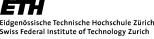Course on

### Satisfiability of Boolean Formulas - Combinatorics and Algorithms

(251-491-00 Erf�llbarkeit logischer Formeln - Kombinatorik und Algorithmen, 2V1U, 5 credits)
Emo Welzl (lecturer) and Andreas Razen (assistant)
Winter 2005/06, Friday, 10-12 (CAB G52, lectures) and 13-14 (CAB G52, exercises)
Language: German, in case nobody expresses preference for English. All accompanying material will be supplied in English.

Contents. Satisfiability (SAT) is the problem of deciding whether a boolean formula in propositional logic has an assignment that evaluates to true. SAT occurs as a problem and is a tool in applications (e.g. Artificial Intelligence and circuit design) and it is considered a fundamental problem in theory, since many problems can be naturally reduced to it and it is the 'mother' of NP-complete problems. Therefore, it is widely investigated and has brought forward a rich body of methods and tools, both in theory and practice (including software packages tackling the problem). This course concentrates on the theoretical aspects of the problem. We will treat basic combinatorial properties (employing the probabilistic method including a variant of the Lovász Local Lemma), recall a proof of the Cook-Levin Theorem of the NP-completeness of SAT, discuss and analyze several deterministic and randomized algorithms and treat the threshold behavior of random formulas. In order to set the methods encountered into a broader context, we will deviate to the more general set-up of constraint satisfaction and to the problem of proper k-coloring of graphs.

Goal. Studying of advanced methods in algorithms design and analysis, and in discrete mathematics along a classical problem in theoretical computer science. Introduction to(wards) a number of up-to-date research topics. Provide basis for independent research on the subject (in a semester/Diplom/master/doctoral thesis).

Prerequisites. The course assumes basic knowledge in propositional logic, probability theory and discrete mathematics, as it is supplied in the Vordiplomstudium.

Literature. There exists no book that covers the many facets of the topic. Lecture notes covering the material of the course will be distributed (Errata, updates, see below).

Here is a list of books with material related to the course. They can be found in the textbook collection (Lehrbuchsammlung) of the Computer Science Library:

George Boole, An Investigation of the Laws of Thought on which are Founded the Mathematical Theories of Logic and Probabilities, Dover Publications (1854, reprinted 1973).
Peter Clote, Evangelos Kranakis, Boolean Functions and Computation Models, Texts in Theoretical Computer Science, An EATCS Series, Springer Verlag, Berlin (2002).
Nadia Creignou, Sanjeev Khanna, Madhu Sudhan, Complexity Classifications of Boolean Constrained Satisfaction Problems, SIAM Monographs on Discrete Mathematics and Applications, SIAM (2001).
Harry R. Lewis, Christos H. Papadimitriou, Elements of the Theory of Computation, Prentice Hall (1998).
Rajeev Motwani, Prabhakar Raghavan, Randomized Algorithms, Cambridge University Press, Cambridge, (1995).
Uwe Schöning, Logik für Informatiker, BI-Wissenschaftsverlag (1992).
Uwe Schöning, Algorithmik, Spektrum Akademischer Verlag, Heidelberg, Berlin (2001).
Michael Sipser, Introduction to the Theory of Computation, PWS Publishing Company, Boston (1997).
Klaus Truemper, Design of Logic-based Intelligent Systems, Wiley-Interscience, John Wiley & Sons, Inc., Hoboken (2004).

Previous Years. web-page course 2003, web-page course 2004.
A Course Schedule.
[28 Oct] (For an exception, lectures extend to the exercises in the afternoon.)
Material covered: Introduction, examples (circuit verification, map labeling), conjunctive normal form, terminology, (3hrs).
Exercises "handed out": 1.3, 1.6, 1.12, 1.17, 1.18.
[4 Nov] Material covered: Counting satisfying assignments, resolution.
Exercises "handed out": 1.20, 1.21, 1.25, 1.29.
[11 Nov] Material covered: Extremal properties (number of clauses/of dependent clauses).
Exercises "handed out": 2.1, 2.2, 2.4.
[18 Nov] Material covered: Partial satisfaction, 2-SAT (resolution, unit clause reduction).
Exercises "handed out": 2.9, 2.10, 2.18, 3.3.
[25 Nov] Material covered: 2-SAT (random walk, implication graph).
Exercises "handed out": 3.6, 3.11, 3.12, 3.13.
[2 Dec] Material covered: 2-SAT applications, SAT and NP (coloring vs SAT).
Exercises "handed out": 3.17, 3.18, 3.20, 4.2.
[9 Dec] Material covered: SAT and NP (polynomial verifiers and k-falsifiers, class NP).
Exercises "handed out": 4.4, 4.9.
[16 Dec] Material covered: SAT and NP (class NP and relatives FPk, polynomial reductions).
Exercises "handed out": 4.5, 4.10.
[23 Dec] Material covered: SAT and NP, The Cube (SAT is NP-complete, Introduction to the n-dim. hypercube).
Exercises "handed out": 2.3, 5.1, 5.4, 5.6.
---(X-mas break)---
[13 Jan] Material covered: The Cube (faces, packing and covering, Kraft Inequality, degree condition for induced subgraphs of cubes, volume of Hamming balls).
Exercises "handed out": 5.9, 5.10, 5.11, 5.12.
[20 Jan] Material covered: The Cube (volume of Hamming balls, encoding satisfying assignments).
Exercises "handed out": 5.14, 5.17.
[27 Jan] Material covered: Encoding satisfying assignments, Paturi-Pudlák-Zane Algorithm (randomized), Hamming balls and k-SAT algorithms.
Exercises "handed out": 6.3, 6.4, 7.2.
[3 Feb] Material covered: Schöning's Algorithm, Random walks.
Exercises "handed out": .
[10 Feb] Material covered: Constraint Satisfaction Problem (CSP).
---(end of semester)---
[Monday 13 Mar, 09:00-11:00 @ HG D1.1] Exam: Check out previous years exams, (February 2004 .pdf or .ps, February 2005 .pdf or .ps). See below for further information.

Lecture Notes. Lecture notes are distributed during the course, including the exercises that we suggest to work through. There is no on-line accessible version of the lecture notes.

Errata:

• Page 28, line -2: ''sc(F)'' should be ''sc(F,\alpha)''.
• Page 73, line 10: ''such that G' is satisfiable iff G is satisfiable'' should be ''such that G' is satisfiable iff Formula(w) is satisfiable''.
• Page 76, line -7 (above footnote): ''ought to be be read'' should be ''ought to be read''.
• Page 105, line -5: ''in a $(\leq k)$-CNF formula'' should be ''in a satisfiable $(\leq k)$-CNF formula''.
• Page 111, line -1: ''will now we improved'' should be ''will now be improved''.
• Page 122, line -11: ''Papdimitriou's'' should be ''Papadimitriou's''.
• Page 124, Figure 8.1: The 'x' in the lower-left set should be inside the quadrangle instead of being inside the circle.
• Page 134, line 10: ''with d_H(\alpha_i,\alpha)'' should be ''with d_H(\alpha_i,\alpha)=1''.
• Page 134, line -6: ''x with \alpha(x) = 0'' should be ''x with \alpha_1(x) = 0''.

Exam: There is a written 2 hours exam that takes place on the 13th of March 2006, from 09:00 to 11:00 at room HG D1.1 , also for the students from D-MATH. You are allowed to take any kind of written material with you, but no electronic devices of whatever kind.

Anybody other than D-INFK students should indicate participation in the exam by email both to Emo Welzl and Andreas Razen before end of January. (D-INFK students are assumed to have subscribed for the exam through the usual procedures.)

Material relevant for the exam is as given in the lecture notes handed out, Chapters 1 through 8 and Glossary of Notions and Facts, with the only exception of the symmetrization proof of Theorem 2.5, but with its proof given in Note 2.5 instead. Note that Chapter 9 which was handed out is not required for the exam.